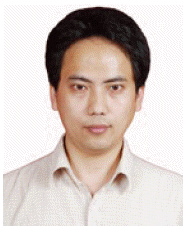Sparse Channel Estimation of Single Carrier Frequency Division Multiple Access Based on Compressive Sensing

Article information

Journal of Information Processing Systems. 2015;11(3):342-353
Publication date (electronic) : 2015 May 21
doi : https://doi.org/10.3745/JIPS.03.0028
*School of Communication Engineering, Chongqing University, Chongqing, PR China (zhongyh@cqu.edu.cn, zyhuang@cqu.edu.cn, zhubin@cqu.edu.cn, wuhua@cqu.edu.cn)
Corresponding Author: Yuan-Hong Zhong (zhongyh@cqu.edu.cn)
Received 2013 December 30; Revised 2014 May 12; Revised 2014 July 28; Accepted 2014 September 01.

Abstract

It is widely accepted that single carrier frequency division multiple access (SC-FDMA) is an excellent candidate for broadband wireless systems. Channel estimation is one of the key challenges in SC-FDMA, since accurate channel estimation can significantly improve equalization at the receiver and, consequently, enhance the communication performances. In this paper, we study the application of compressive sensing for sparse channel estimation in a SC-FDMA system. By skillfully designing pilots, their patterns, and taking advantages of the sparsity of the channel impulse response, the proposed system realizes channel estimation at a low cost. Simulation results show that it can achieve significantly improved performance in a frequency selective fading sparse channel with fewer pilots.

1. Introduction

In a typical wireless scenario, the transmitted signal arrives at the receiver via various paths of different lengths. This leads to inter-symbol interference (ISI) and creates a major difficulty for information decoding. Furthermore, using the traditional single carrier modulation with the time domain equalization approach is unattractive for wide-band system due to the high complexity of the receiver. Instead, the frequency domain equalization technology is a good choice for wide-band wireless communication system (e.g., for orthogonal frequency division multiplexing (OFDM) ). OFDM has been widely applied in wireless communication systems because of its high bandwidth efficiency and relative robustness against multipath fading and delay. However, it suffers from a large peak to average power ratio (PAPR).

On the other hand, single carrier modulation with frequency domain equalization, which attempts to approach the performance and complexity of OFDM while maintaining a lower PAPR as compared to OFDM, is another excellent technology for broadband systems. Single carrier frequency division multiple access (SC-FDMA)  is the multiple access form of single carrier modulation with frequency domain equalization Lately it has received a lot of attention and has become an alternative to orthogonal frequency division multiple access for 4G technologies. SC-FDMA has been adopted for the uplink transmission technique in both 3GPP Long Term Evolution (LTE) and LTE Advanced standards . Since most of the cost in communication terminals comes from the power amplifier, a lower PAPR, which is the advantage of SC-FDMA, can significantly reduce the cost of mobile units. This results in more power efficient and less complex mobile terminals. Since the orthogonal frequency division multiple access (OFDMA) is used in the downlink, both the burdens of the complex frequency domain equalizer needed for the SC-FDMA and accommodating large PAPRs in OFDMA rest upon the base station.

Channel estimation is critical to the performance of coherent SC-FDMA demodulation; channel estimation, which is also related to diversity reception; the optimum match receiver design; and adaptive link technologies. All of these technologies require good channel estimation support. Therefore, accurate channel estimation can significantly improve the performance of the SC-FDMA system [4,5]. However, numerous experimental studies undertaken by various researchers in the recent past have shown that wireless channels associated with a number of scattering environments tend to exhibit sparse structures in the sense that majority of the channel taps end up being either zero or below the noise floor when operating at large bandwidths and symbol durations and/or with a large plurality of antennas . However, traditional training-based methods that rely on linear reconstruction schemes at the receiver seem incapable of exploiting the inherent low dimensionality of such sparse channels, thereby leading to overutilization of the key communication resources of energy, latency, and bandwidth. A number of researchers have tried to address this problem in the recent past and have proposed training signals and reconstruction strategies that are tailored to the anticipated characteristics of sparse multipath channels . Recently, there has been a growing interest in compressed sensing (CS) , which has been widely applied in many areas such as image processing, communication systems, and so on. According to the CS theory, if a signal has a sparse representation in a certain space, one can sample the signal at a rate that is significantly lower than the Nyquist rate and reconstruct it with high probability by applying optimization techniques. The essence of pilot assisted channel estimation in OFDM systems is to reconstruct the channel frequency response from pilot symbols. Hence, it is natural to consider using the CS theory in pilot-assisted sparse channel estimation to reduce the number of pilot symbols. In , the CS theory has been employed for sparse channel estimation in OFDM systems. However, litter work has been found in the SC-FDMA system. This paper will investigate the application of compressive sensing in the SC-FDMA system.

The rest of paper is organized as follows: in Section 2 we review the general SC-FDMA system model and give the problem statement. In Section 3 we present compressive sensing and pilot design in SC-FDMA. Section 4 introduces several recovery methods of compressive sensing and analyzes its complexity. Finally, Section 5 presents the simulation results and concludes our work.

2. Problem Statement

2.1 SC-FDMA

Fig. 1 depicts the high level model of an SC-FDMA receiver and transmitter [2,3]. M modulated source symbols are converted to frequency domain. The frequency domain symbols are then mapped onto M out of N (M<N) possible orthogonal subcarriers. Subcarriers can be mapped in the following two ways: localized mapping, where each user is assigned a set of m consecutive subcarriers; and distributed mapping, where subcarriers assigned to the user are equally spaced across the entire channel bandwidth. After converting the symbols back to the time domain using an N-point IDFT and inserting the cyclic prefix, the SC-FDMA time domain symbol is transmitted through the channel. At the receiver all of the steps are reversed. The crucial difference between the SC-FDMA and OFDMA comes from the additional DFT block before subcarrier mapping. The DFT block ‘spreads’ the modulated source symbols, so that each subcarrier in the frequency domain contains information about all the source symbols.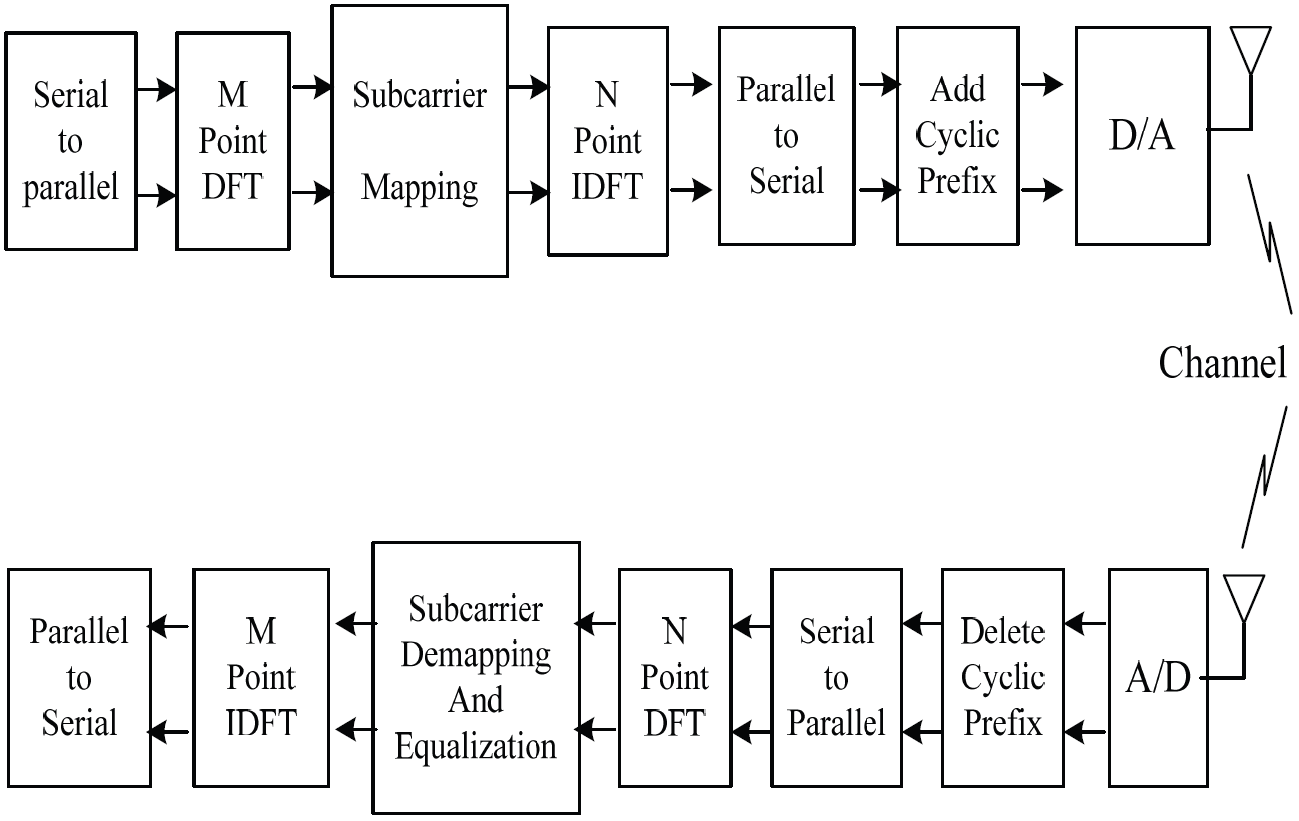System model of single carrier frequency division multiple access (SC-FDMA). IDFT=inverse discrete Fourier transform.

2.2 Formulation of Channel Estimation

Let’s consider a frequency selective fading channel model whose coherence time is much larger than the SC-FDMA symbol duration and the multi-path delays are sampled equispaced. Then, the multipath channel can be modeled as a finite impulse response (FIR) filter:

(1) h(n)=l=0L-1hlδ(n-l)

where L is the total number of taps and hl is the l th tap’s complex gain. Assume that the channel is sparse, which means the vector h = [h0, h1,…, hL−1]T has only a few nonzero elements; but the location of the nonzero taps is not determined.

To simplify the analysis, let’s consider one user. Suppose that the SC-FDMA system consists of N sub-carriers amongst which K sub-carriers are reserved for pilot symbols, as shown in Fig. 2. The pilot inserting method is known as the Frequency Expanding Technique . Generally, a comb-pilot pattern is used, as shown in Fig. 3, and the length of the cyclic prefix (CP), LCP, is not less than L.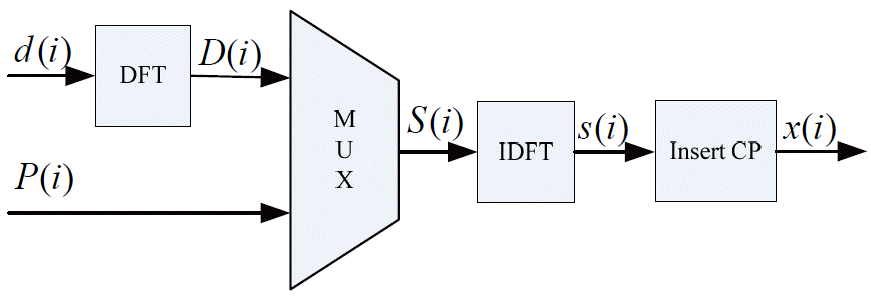Insertion method of pilot data. IDFT=inverse discrete Fourier transform, CP=cyclic prefix.

At the receiving end, after the CP has been synchronized and deleted, it is convenient to get a matrix formulation of an SC-FDMA system as:

(2) Y=XH+W=XFh+W

where Y is the reception block signal in the frequency domain of length N, and X = diag(S0, S1,…SN−1), HCNX1 is the frequency domain representation of h, and FCNXL is the FFT matrix:

(3) F=1N[f00f(L-1)0f0(N-1)f(L-1)(N-1)]

where fnl=e-2πjnlN, and W is the white Gaussian noise signal in the frequency domain.

3. Sparse Channel Estimation Based on Compressive Sensing

3.1 Review of Compressed Sensing

The CS theory  has recently become widely popular for improving system efficiency in the signal processing community, such as video coding, compressed image and sensors. We will now provide a brief review of sparse signal reconstruction with the CS theory.

Generally, signal processing problems are mapped into a linear observation model consisting of some linear transformation of a sparse vector, such as:

(4) y=Φh

where Φ ∈ RKXL, while h is S-sparse (i.e., no more than s of its elements are nonzero). Obviously, if K< L or rank(Φ)< L, the system of equations is underdetermined and the solution is not unique. However, we have the additional knowledge that h is sparse. Thus, we search over the solution space for the sparsest solution, as shown in the following optimization problem:

(5) h=argminh0,s.t.y=Φh

where ||h||0 is the mean operation of the 0-norm, which is defined to be the number of nonzero entries in h. This optimization problem is generally combinatorial in nature. A number of authors [16,17] have proposed a more tractable relaxation of it, namely:

(6) h=argminh1,s.t.y=Φh

where ||h||1 is the mean operation of the L1-norm of h. This is a convex optimization problem that can be solved using the standard algorithms basis pursuit (BP) . Eq. (5) is equivalent to Eq.(6) which has a unique solution, and the measurement matrix Φ, after scaling, needs to satisfy the restricted isometry property (RIP) [17,18]:

(7) (1-δS)h22Φh22(1+δS)h22

where ||h||2 is the mean operation of the L2-norm of h. For all S-sparse vectors hRL, for any δS ∈ (0,1), and any c1 < δS (3 −δS) 48, set c2=192log(12/δS)/(3δS2-σS3-48c1). A satisfaction of RIP with the order S and parameter δS can have a probability of at least 1−ec1K to recover h, whenever Kc2 Slog L . The RIP is satisfied with high probability by a large class of random matrices, such as those with entries independently sampled from a sub-Gaussian distribution. The works in [19, 20] also study the stable recovery from noisy observations based on conditions of δS. In the presence of noise, the signal reconstruction can be obtained as:

(8) h=argminh1,s.t.Φh-y2ɛ

Solving the optimization problem in Eq. (6) is computationally expensive and is not suitable for real-time applications. Faster and more efficient reconstruction algorithms that use iterative greedy-based algorithms at the expense of slightly more measurements, such as matching pursuit , orthogonal matching pursuit (OMP) , and CoSaMP , exist.

3.2 Measurement Matrix and the Pilot Pattern Design

According to Section 3.1, designing the measurement matrix Φ with RIP would lead to the stable recovery of sparse signals h from noisy observations y. In  the system is modeled in the time domain directly in terms of the channel coefficients. Therefore, to identify the frequency-selective channel, it is becomes equivalent to designing the (discrete) input probe of the time-domain Toeplitz convolution matrix, which satisfies RIP. The channel coefficients are estimated from Toeplitz channel measurements. However, the Toeplitz convolution matrix increases the computational complexity.

In this paper, a random pilot matrix with RIP is presented to reconstruct the sparse channels. According to , measure matrices that satisfy RIP(2S,2-1) include random Gaussian, Bernoulli, and partial Fourier matrices. Therefore, we can induce the measure matrices from formula (2) with these matrices. Let QCKXN be a selection matrix that selects the elements on the pilot locations from an N-dimensional vector. Specifically, Q can be generated by selecting K rows from a NXN unit matrix. Then, the received pilot symbols are given by:

(9) YP=XPFPh+WP

where YP = QY, XP = QXQH, FP = QF, Wp = QW and YPCKX1, XPCKXK, FPCKXL, WpCKX1. Here, YP, XP and FP are all known to the receiver. Commonly, the coefficients of the channel are estimated directly (i.e., if KL, the vector h is invertible). This is a simple linear estimator in the observations where the least squares (LS)  channel estimator is given by:

(10) h^ls=(FPHFP)-1FPHXP-1YP

If K< L, the performance of LSE will be poor, especially when h is a sparse vector the estimated performance will be very bad for not using the sparse property. In this case, the LS channel estimator is given by:

(11) h^ls=FPH(FPFPH)-1XP-1YP

According to Eq. (9), it is known that this is the compressive sensing system model that was introduced in Section 3.1, where the measurement matrix is XPFP, which is determined by the pilot symbols and pilot locations. If XPFP satisfy the RIP, then we can solve it with a compressive sensing recovery algorithm such as BP, OMP, lasso, and so on. With Q generated by selecting K rows from a NXN unit matrix randomly, the channel is probed randomly with the random sequence {P(i)} in the frequency domain. {P(i)} are i.i.d. realizations from a zero mean, unit variance distribution, and P(i) ’s take values are +1 or –1 with a probability of 1/2 each. The positions in the input probe in this case match with the matrix Q, where Kc2Slog L satisfies the RIP of order S with the parameter δS with the probability being at least 1−ec1K. The insertion method for random pilots is demonstrated in Fig. 3.

3.3 Performance Analysis

Assuming the noise variance is deterministic, it is possible to obtain a reliable estimator of h as a solution to the convex program. Let Φ = XPFP be an observation matrix satisfying RIP of order 2S such than δ2S<2-1 for some integer S ≥ 1. Also, let Y = Ah+W be a vector of noisy observations of h, where ||W||2 < ɛ. The solution of :

(12) h^=argminh^1,   s.t.Φh-y2ɛ

will obey:

(13) h^-h2c0(ɛ+hS-h1S)2

where hS is the best s-sparse approximation to h; the nonzero components in hS are the s largest components of h, and c0=16(1+δ2S)/(1-δ2S-2δ2S)2.

Let SK be the set of indices of S nonzero entries of h, which can be termed the channel sparsity pattern of s-sparse channel. Assume that the channel sparsity pattern and the corresponding virtual coefficients h are deterministic but unknown. Then Φ̃SK is a submatrix obtained by extracting the position of observation matrix Φ corresponding to the indices in SK and the mean square error (MSE) of the proposed channel estimator can be low bounded as [19,25]:

(14) MSE=E{h^-h22}=σ2tr{(Φ˜SKHΦ˜SK)-1}σ2Sγlog(L)

where σ2 is the zero-mean white Gaussian with variance, γ is the pilot symbol energy, and tr(.) denotes the trace operator. The performances of the proposed channel estimator are better than that of the LS channel estimator with the MSE as σ2Lγ.

4. Simulation Results

In this section, Monte Carlo simulations are conducted to show the channel estimation performance of the proposed algorithm. To begin with, assume that a symbol block in the system of SC-FDMA is time-invariant, the length of the symbol block is N = 512, the maximum channel length is L = 64, and the number of pilot is K. The multi-path channel has the characteristics of negative exponential power delay. That is, each path power is δl2 = exp(−4l/Ncp), l = 0,1,…L −1, where Ncp = 64 is the length cyclic prefix; the number of nonzero channel taps V = 4; and the system is synchronized to the first path. This means that h0 is always nonzero, and other path delays are randomly distributed over the entire channel length of L.

In order to evaluate the performance of the channel estimation algorithm, we defined the channel estimation of the normalized mean square error (NMSE) as follows:

(15) NMSE=E{kHk-H^k2}E{kHk2}

In fact, the direct assessment in the frequency domain is mainly because the SC-FDMA system implements equalization in the frequency domain.

First, in order to evaluate the effectiveness of CS algorithms, we compared the MSE of LS and CS with a different recovery algorithm under a different pilot. For the LS estimator, the comb-type pilot arrangement can make the channel estimation optimal; therefore, the comb-pilot is used. For CS algorithms random pilots are used. Figs. 4 and 5 show the MSE performance of LS and CS under the pilot numbers K = 16 and K = 24 . As can be seen from the figure, when the LS algorithm uses a small number of pilots, (i.e., K< L ), the algorithm is basically in an invalid state and the system would have serious errors. That’s because the LS channel estimation method requires the insertion of a pilot number that is not lower than the actual channel length, which requires KL = 64. In Figs. 4 and 5, the performance of LS with pilot number K = 64 is also given. It is evident that the LS algorithm improves greatly when KL.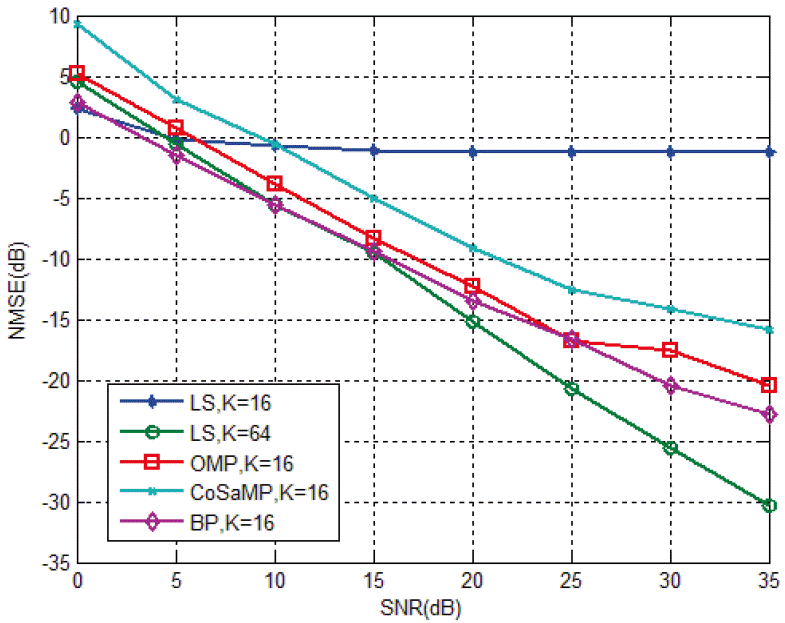Normalized mean square error (NMSE) performance of least square (LS) and compressed sensing, pilot number K=16. SNR=signal-to-noise ratio, OMP=orthogonal matching pursuit, CoSaMP= compressive sampling matching pursuit, BP=basis pursuit.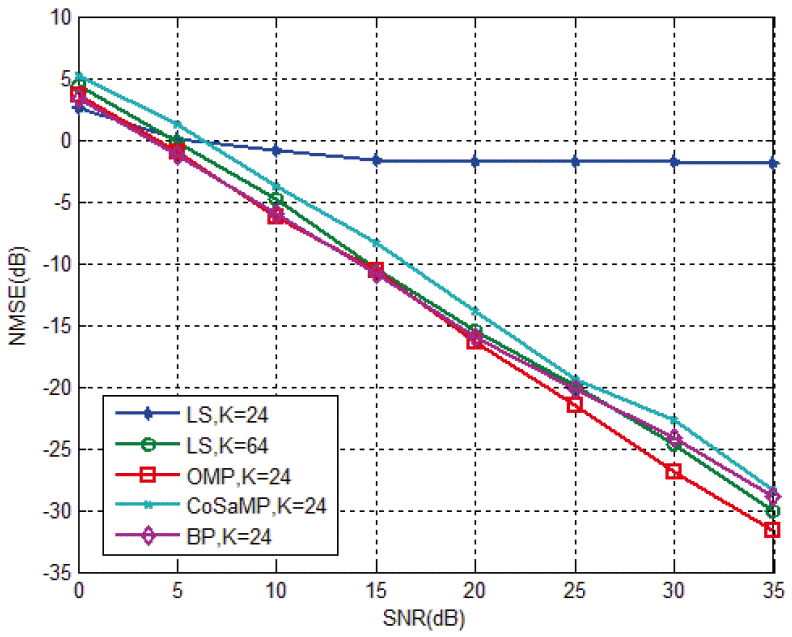Normalized mean square error (NMSE) performance of least square (LS) and compressed sensing, pilot number K=24. SNR=signal-to-noise ratio, OMP=orthogonal matching pursuit, CoSaMP= compressive sampling matching pursuit, BP=basis pursuit.

In addition, we can see from Figs. 4 and 5 that the three CS-based recovery algorithms all have a better performance when a small number of pilots are used. Moreover, the OMP algorithm performs better than BP and CoSaMP does. The OMP algorithm uses a fast iterative mode to achieve better speed than BP. Although the calculation amount of CoSaMP is also small, its performance is quite different with the other two algorithms. Therefore, in a real system application, the OMP algorithms can be considered for recovering the channel response for their low complexity. Even more importantly, the need of the pilot is very small. Therefore, the transmission efficiency of the system can be improved greatly.

As shown in Figs. 4 and 5, we can also see that the recovery performance of the algorithm depends on the number of pilots. Therefore, we simulated the CS recovery performance of the algorithm under a different number of pilots in order to study the relationship between them. Fig. 6 shows the NMSE curve of channel estimation with a different number of pilots and the SNR of the SC-FDMA system is fixed at 15 dB. From Fig. 6 it can be seen that the estimated performance of LS is poor when the pilot is less than the channel length. When it is only close to or greater than the channel length, it has better performance; and when the pilot number is more than the channel length, the increase in performance is not obvious. For the CS recovery algorithms, a small number of pilots can get a better performance as compared to LS. However, when the pilot number is greater than about 20, the increase in performance is not very obvious. Based on our experience, the recommended number of pilots is 4–5 times the number of nonzero channel taps. Therefore, there is a tradeoff between the system transfer efficiency and performance.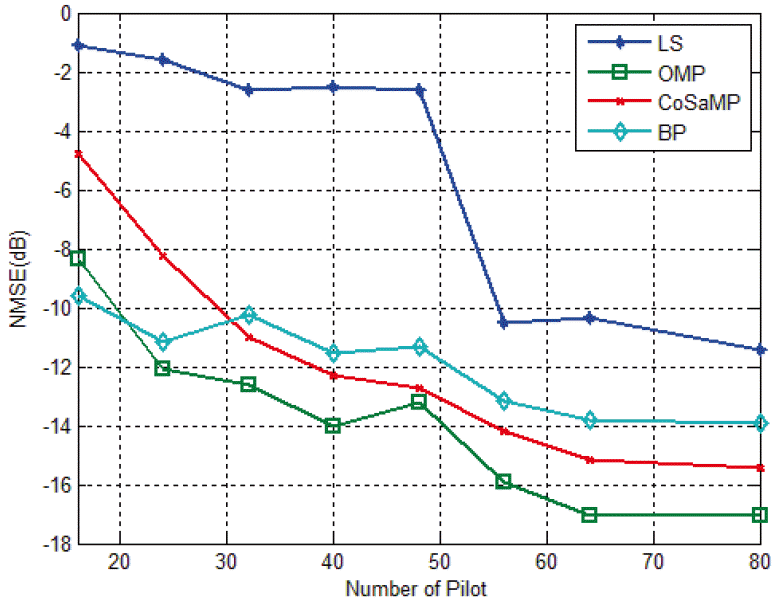Normalized mean square error (NMSE) performance of different number of pilots. LS=least square, OMP=orthogonal matching pursuit, CoSaMP=compressive sampling matching pursuit, BP=basis pursuit.

Finally, the impact of pilot arrangement on system performance is evaluated in Fig. 7. For the LS estimation, a comb-type pilot arrangement is used, and it has the best performance. Whereas, with regard to the CS algorithm, the sensor matrix needs to meet RIP constraint as we have already discussed. Therefore, a random arrangement pilot is used, as shown in Fig 3. For the sake of contrast, the NMSE of CS when using the comb-type pilot is also shown, and the pilot number K = 24 is selected. Fig. 7 shows the performance comparison of the two arrangements. It can be seen that randomly arranging the pilot results in better performance. However, randomly selecting the pilot brings about the increasing of complexity. In practice, the receiver needs to store the random pilot pattern, which increases the system storage capacity. In  the optimal pilot pattern is designed by minimizing the mutual coherence of the measurement matrix. This idea can be borrowed to further improve the performance of estimation.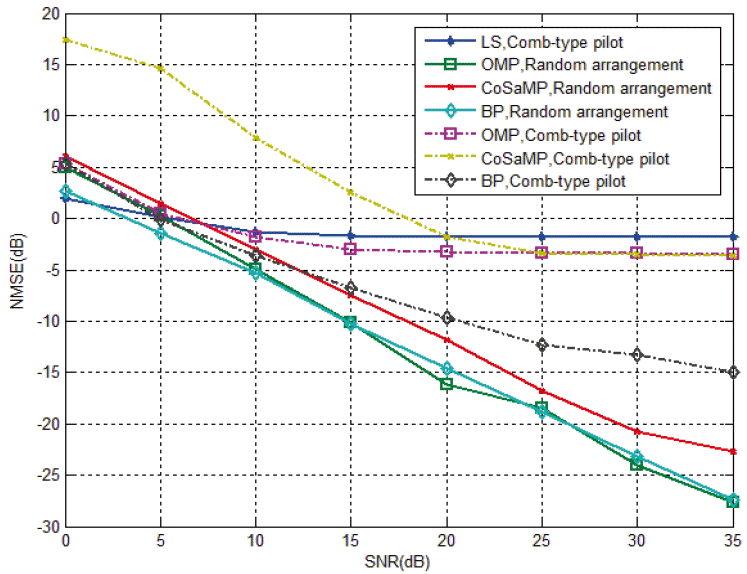Performance comparison of different pilot-insertion methods. NMSE=normalized mean square error, SNR=signal-to-noise ratio, LS=least square, OMP=orthogonal matching pursuit, CoSaMP=compressive sampling matching pursuit, BP=basis pursuit.

5. Conclusion

In this paper, a CS based channel estimation algorithm is presented for the sparse channel in the SC-FDMA system. The proposed scheme, using a novel random pilot matrix pattern and the CS algorithm, has lower NMSE with fewer pilots. The analysis and simulation results verify that the proposed algorithm is effective in improving the system utilization of the spectrum. The algorithm can be applied to a wireless SC-FDMA system, such as LTE, with no extra architectural changing.

Acknowledgement

This work was supported in part by the Fundamental Research Funds for the Central Universities of China under Grant No. CDJZR12160015 and No. CDJZR12160005, and also supported by Key Program of Natural Science Foundation of Chongqing under Grant No. CSTC2013JJB40004, China.

References

1. Proakis JG, Salehi M. Digital Communication 5th edth ed. Dubuque, IA: McGraw-Hill; 2007.
2. Myung HG, Lim J, Goodman D. Single carrier FDMA for uplink wireless transmission. IEEE Vehicular Technology Magazine 1(3):30–38. 2006;
3.

3GPP TS 36.201 version 11.1.0, LTE Physical Layer - General description (Release 11), 2013

4. Karakaya B, Arslan H, Cirpan HA. Channel estimation for LTE uplink in high Doppler spread. In : Proceedings of IEEE Wireless Communications and Networking Conference (WCNC 2008). Las Vegas, NV; 2008. p. 1126–1130.
5. Al-kamali FS, Dessouky MI, Sallam BM, Shawki F, El-Samie FA. Uplink single-carrier frequency division multiple access system with joint equalisation and carrier frequency offsets compensation. IET Communications 5(4):425–433. 2011;
6. Cotter SF, Rao BD. Sparse channel estimation via matching pursuit with application to equalization. IEEE Transactions on Communications 50(3):374–377. 2002;
7. Carbonelli C, Vedantam S, Mitra U. Sparse channel estimation with zero tap detection. IEEE Transactions on Wireless Communications 6(5):1743–1763. 2007;
8. Bajwa WU, Haupt J, Sayeed AM, Nowak R. Compressed channel sensing: a new approach to estimating sparse multipath channels. Proceedings of the IEEE 98(6):1058–1076. 2010;
9. Berger CR, Wang Z, Huang J, Zhou S. Application of compressive sensing to sparse channel estimation. IEEE Communications Magazine 48(11):164–174. 2010;
10. Donoho DL. Compressed sensing. IEEE Transactions on Information Theory 52(4):1289–1306. 2006;
11. Zhou X, Fang Y, Wang M. Compressed sensing based channel estimation for fast fading OFDM systems. Journal of Systems Engineering and Electronics 21(4):550–556. 2010;
12. Meng J, Yin W, Li Y, Nguyen NT, Han Z. Compressive sensing based high-resolution channel estimation for OFDM system. IEEE Journal of Selected Topics in Signal Processing 6(1):15–25. 2012;
13. Taubock G, Hlawatsch F. A compressed sensing technique for OFDM channel estimation in mobile environments: exploiting channel sparsity for reducing pilots. In : Proceedings of IEEE International Conference on Acoustics, Speech and Signal Processing (ICASSP 2008). Las Vegas, NV; 2008. p. 2885–2888.
14. He X, Song R, Zhu WP. Optimal pilot pattern design for compressed sensing-based sparse channel estimation in OFDM systems. Circuits, Systems, and Signal Processing 31(4):1379–1395. 2012;
15. Lam CT, Falconer DD, Danilo-Lemoine F, Dinis R. Channel estimation for SC-FDE systems using frequency domain multiplexed pilots. In : Proceedings of 2006 IEEE 64th Vehicular Technology Conference (VTC-2006 Fall). Montreal, Canada; 2006. p. 1–5.
16. Chen SS, Donoho DL, Saunders MA. Atomic decomposition by basis pursuit. SIAM Journal on Scientific Computing 20(1):33–61. 1998;
17. Candes EJ. The restricted isometry property and its implications for compressed sensing. Comptes Rendus Mathematique 346(9):589–592. 2008;
18. Candès EJ, Romberg J, Tao T. Robust uncertainty principles: exact signal reconstruction from highly incomplete frequency information. IEEE Transactions on Information Theory 52(2):489–509. 2006;
19. Donoho DL, Elad M, Temlyakov VN. Stable recovery of sparse overcomplete representations in the presence of noise. IEEE Transactions on Information Theory 52(1):6–18. 2006;
20. Tropp JA. Just relax: convex programming methods for identifying sparse signals in noise. IEEE Transactions on Information Theory 52(3):1030–1051. 2006;
21. Mallat SG, Zhang Z. Matching pursuits with time-frequency dictionaries. IEEE Transactions on Signal Processing 41(12):3397–3415. 1993;
22. Tropp JA, Gilbert AC. Signal recovery from partial information via orthogonal matching pursuit Apr. 2005. http://www.math.lsa.umich.edu/~annacg/papers/TG05-Signal-Recovery.pdf.
23. Needell D, Tropp JA. CoSaMP: iterative signal recovery from incomplete and inaccurate samples. Applied and Computational Harmonic Analysis 26(3):301–321. 2009;
24. Kay SM. Fundamentals of Statistical Signal Processing: Estimation Theory Englewood Cliffs, NJ: Prentice-Hall; 1998.
25. Sharp M, Scaglione A. Estimation of sparse multipath channels. In : Proceedings of IEEE Military Communications Conference (MILCOM 2008). San Diego, CA; 2008. p. 1–7.

Biography

Yuan-Hong Zhong

He received his B.S., M.S., and Ph.D. degrees in communication engineering from Chongqing University, Chongqing, China in 2003, 2006, and 2011, respectively. And now he works in the School of Communication Engineering at Chongqing University. His research interests include wireless communication, wireless multimedia sensor network, and computer vision.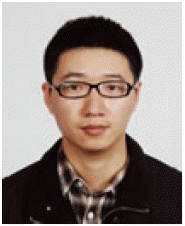Zhi-Yong Huang

He received his Ph.D. degree in circuit and system from Chongqing University, in 2010. He is currently an associate professor in the School of Communication Engineering at Chongqing University. His research interests are in the areas of network security, communication system and control system.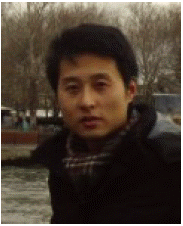Bin Zhu

He received his Ph.D. degree in communication and information system from Chongqing University, in 2010. He is currently an associate professor in the School of Communication Engineering at Chongqing University. His research interests include in the areas of wireless sensor network, communication system and wireless communication network.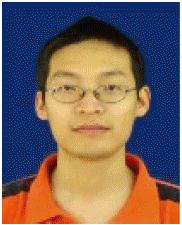Hua Wu

He received his Ph.D. degree in communication and information system from Chongqing University, in 2009. And now He works in the School of Communication Engineering at Chongqing University. His research interests include wireless communication, filter bank and computer vision.